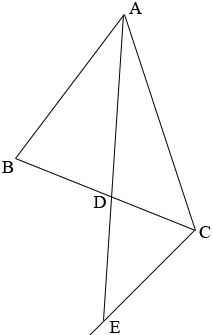SEARCH HOMEMath Central Quandaries & QueriesQuestion from ramarao, a parent: D is a point on side BC of a triangle ABC such that BD/CD=AB/AC. prove that AD is the bisector AD is the bisector of BACRamarao,

This is a standard result that can be easily found in textbooks. There are two proofs in cut-the-knot

http://www.cut-the-knot.org/Curriculum/Geometry/AngleBisectorRatio.shtml

but both are harder than they have to be, and the converse (which is needed here) is consequently much harder. So here's my version.

GIVEN: D is a point on the side BC of triangle ABC such that BD/CD = AB/AC.
TO PROVE: AD bisects <BAC (that is, <BAD = <DAC).Draw the parallel to AB through C and call E the point where it meets the extension of AD. Then <CED = <BAD (alternate interior angles); moreover the angles at D are equal so that triangles BAD and CED are similar. Consequently,

AB/EC = BD/CD.

But we are given that BD/CD = AB/AC, whence EC = AC. Because we have in triangle ACE that CE=CA, it follows that the base angles at A and E are equal; that is, <DAC = <CED , which equals <BAD, as desired.

The converse (if AD bisects <BAC then AB/AC = BD/CD) can be proved by reversing the above argument.

ChrisMath Central is supported by the University of Regina and The Pacific Institute for the Mathematical Sciences.